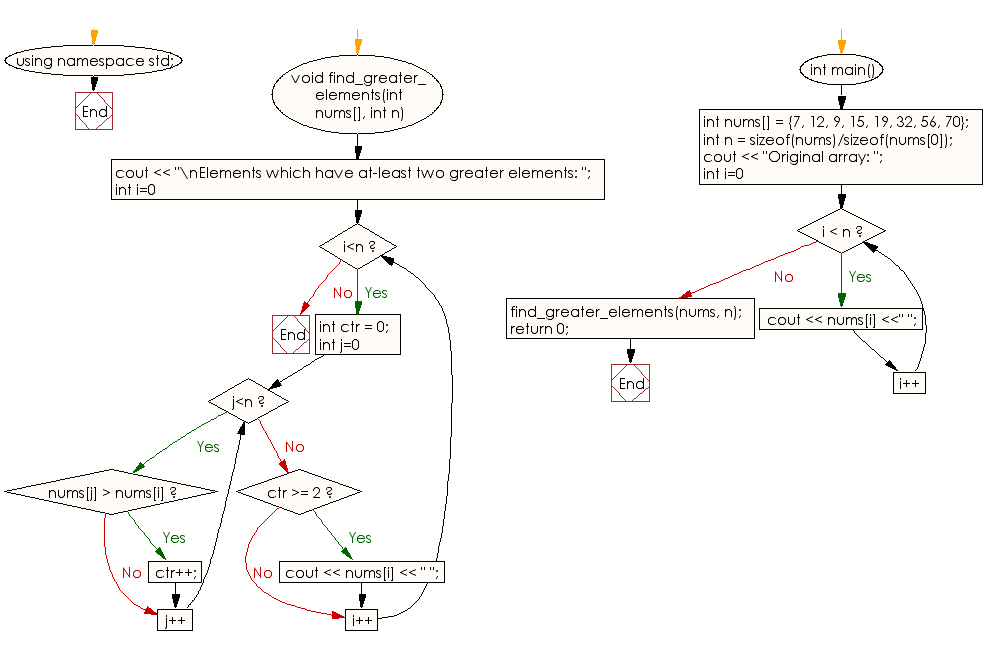﻿ C++ : Elements in an array which have at-least two greater# C++ Exercises: Find all elements in array of integers which have at-least two greater elements

## C++ Array: Exercise-6 with Solution

Write a C++ program to find all elements in array of integers which have at-least two greater elements.

Sample Solution:

C++ Code :

``````#include<iostream>
using namespace std;

void find_greater_elements(int nums[], int n)
{
cout << "\nElements which have at-least two greater elements: ";
for (int i=0; i<n; i++)
{
int ctr = 0;
for (int j=0; j<n; j++)
if (nums[j] > nums[i])
ctr++;

if (ctr >= 2)
cout << nums[i] << " ";
}
}

int main()
{
int nums[] = {7, 12, 9, 15, 19, 32, 56, 70};
int n = sizeof(nums)/sizeof(nums);
cout << "Original array: ";
for (int i=0; i < n; i++)
cout << nums[i] <<" ";
find_greater_elements(nums, n);
return 0;
}
``````

Sample Output:

```Original array: 7 12 9 15 19 32 56 70
Elements which have at-least two greater elements: 7 12 9 15 19 32
```

Flowchart:C++ Code Editor:

What is the difficulty level of this exercise?

Test your Programming skills with w3resource's quiz.

﻿